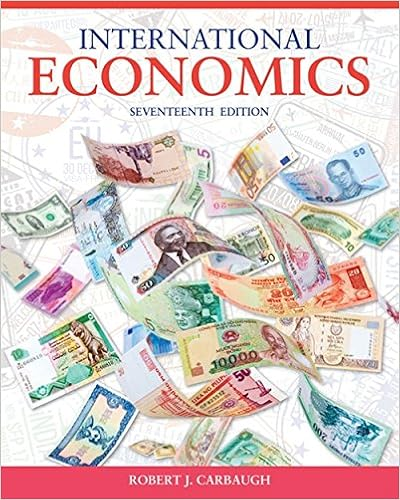# 66 using risk neutral valuation ie the binomial

• Notes
• khaehee
• 73
• 86% (182) 157 out of 182 people found this document helpful

This preview shows page 58 - 66 out of 73 pages.

##### We have textbook solutions for you!
The document you are viewing contains questions related to this textbook.The document you are viewing contains questions related to this textbook.
Chapter 11 / Exercise 7
International Economics
CarbaughExpert Verified
66. USING RISK NEUTRAL VALUATION (i.e. the binomial option pricing model) find the value of the call (in euro). 7-58
##### We have textbook solutions for you!
The document you are viewing contains questions related to this textbook.The document you are viewing contains questions related to this textbook.
Chapter 11 / Exercise 7
International Economics
CarbaughExpert Verified
Chapter 07 Futures and Options on Foreign Exchange67. Calculate the hedge ratio 68. State the composition of the replicating portfolio; your answer should contain "trading orders" of what to buy and what to sell at time zero. 69. Find the value today of your portfolio in euro. 70. If the call finishes out-of-the-money what is your portfolio cash flow? 71. If the call finishes in-the-money what is your portfolio cash flow? 7-59
Chapter 07 Futures and Options on Foreign Exchange72. Use your results from the last three questions to verify your earlier result for the value of the call. Consider an option to buy €12,500 for £10,000. In the next period, the euro can strengthen against the pound by 25% (i.e. each euro will buy 25% more pounds) or weaken by 20%.Big hint: don't round, keep exchange rates out to at least 4 decimal places.73. Calculate the current €/£ spot exchange rate. 7-60
Chapter 07 Futures and Options on Foreign Exchange74. Draw the tree 75. Find the risk neutral probability of an "up" move. 76. USING RISK NEUTRAL VALUATIONfind the value of the call (in euro) 77. Calculate the hedge ratio 7-61
Chapter 07 Futures and Options on Foreign Exchange78. State the composition of the replicating portfolio; your answer should contain "trading orders" of what to buy and what to sell at time zero. 79. Find the cost today of your hedge portfolio in pounds. 80. If the call finishes out-of-the-money what is your portfolio cash flow? 81. If the call finishes in-the-money what is your portfolio cash flow? 82. Use your results from the last three questions to verify your earlier result for the value of the call. 7-62
Chapter 07 Futures and Options on Foreign Exchange83. Find the dollar value today of a 1-period at-the-money calloption on ¥300,000. The spot exchange rate is ¥100 = \$1.00. In the next period, the yen can increase in dollar value by 15 percent or decrease by 15 percent. The risk free rate in dollars is i\$= 5%; The risk free rate in yen is i¥= 1% Multiple Choice Questions7-63
Chapter 07 Futures and Options on Foreign Exchange84. Find the hedge ratio for a put option on \$15,000 with a strike price of €10,000. In one period the exchange rate (currently S(\$/€) = \$1.50/€) can increase by 60% or decrease by 37.5% (i.e. u = 1.6 and d= 0.625). A.-15/49B. 5/13C. 3/2D. 15/497-64
Chapter 07 Futures and Options on Foreign Exchange85. Find the hedge ratio for a put option on €10,000 with a strike price of \$15,000. In one period the exchange rate (currently S(\$/€) = \$1.50/€) can increase by 60% or decrease by 37.5% (i.e. u = 1.6 and d= 0.625). A. -15/49B. 8/13C.-5/13D. 15/49
•••